Skip to content
Related Articles
SQL Query to convert NUMERIC to NVARCHAR
• Last Updated : 15 May, 2021

Here we will see, how to convert NUMERIC data to NVARCHAR data in a MS SQL Server’s database table using the CAST(), CONVERT() and FORMAT() functions.

We will be creating a person table in a database called “geeks”.

### Creating the Database:

`CREATE DATABASE geeks;`

### Using the Database:

`USE geeks;`

### Table Definition:

We have the following Employee table in our geeks database :

```CREATE TABLE person(
id INT IDENTITY(1,1) PRIMARY KEY,
name VARCHAR(30) NOT NULL,
weight NUMERIC(10,5) NOT NULL);
--Here NUMERIC(10,5) says there should be total 10 digits given that 5 digits would be
--to the right of the decimal point.```

You can use the below statement to query the description of the created table:

`EXEC SP_COLUMNS person;`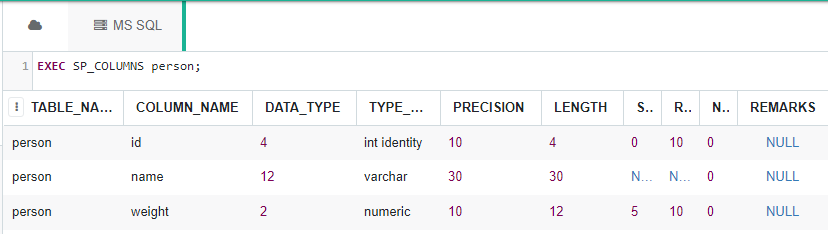### Adding Data to Table:

Use the below statement to add data to the person table:

```INSERT INTO person
VALUES
('Yogesh Vaishnav', 62.5),
('Vishal Vishwakarma', 70),
('Ashish Yadav', 69),
('Ajit Yadav', 71.9);```

To verify the contents of the table use the below statement:

`SELECT * FROM person;`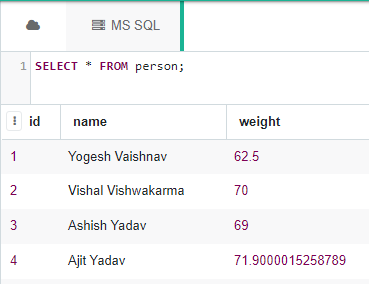Now let’s convert NUMBERIC values to NVARCHAR using three different methods.

• Using the CONVERT() function:
```Syntax: SELECT CONVERT(<DATA_TYPE>, <VALUE>);
--DATA_TYPE is the type we want to convert to.
--VALUE is the value we want to convert into DATA_TYPE.```

Example:

```SELECT 'Weight of Yogesh Vaishnav is ' + CONVERT(NVARCHAR(20), weight)
AS person_weight
FROM person
WHERE name = 'Yogesh Vaishnav';```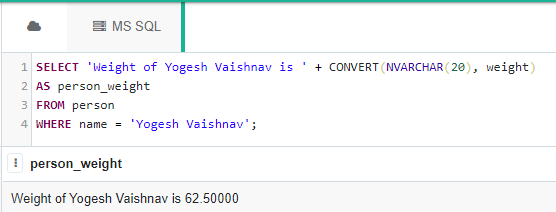As you can see that there are total 5 digits to the right side of the decimal point as discussed above.

• Using the CAST() function:
```Syntax: SELECT CAST(<VALUE> AS <DATA_TYPE>);
--DATA_TYPE is the type we want to convert to.
--VALUE is the value we want to convert into DATA_TYPE```

Example:

```SELECT 'Weight of Ajit Yadav is ' + CAST(weight as NVARCHAR(20))
AS person_weight
FROM person
WHERE name = 'Ajit Yadav';```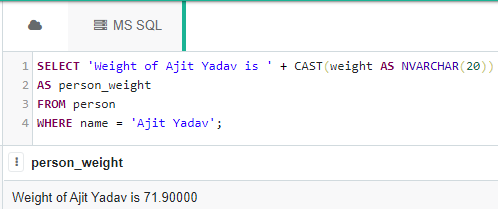• Using the FORMAT() function:

Although FORMAT() function is useful for formatting datetime and not converting one type into another, but still can be used to convert(or here format) float value into an STR value.

```Syntax: SELECT FORMAT(<VALUE> , 'actual_format';
--actual_format is the format we want to achieve in a string form.
--VALUE is the value we want to format according to the actual_format.```

Example:

```SELECT 'Weight of Ashish Yadav is ' + FORMAT(weight, '') --'' denotes no formating
--i.e simply convert it to a string of characters.
AS person_weight
FROM person
WHERE name = 'Ashish Yadav';```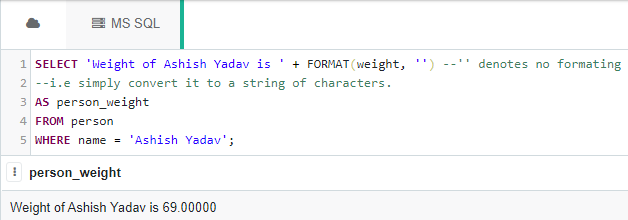My Personal Notes arrow_drop_up